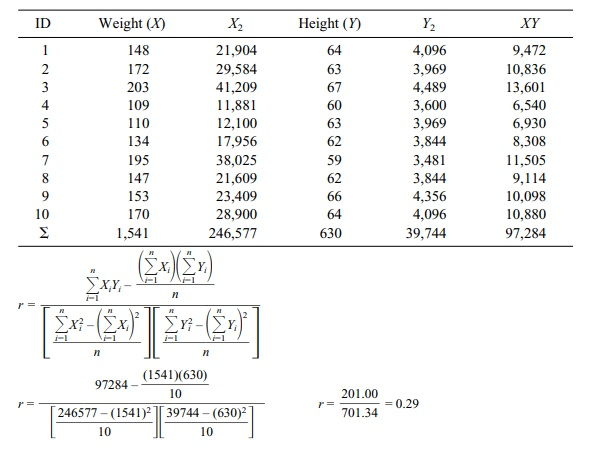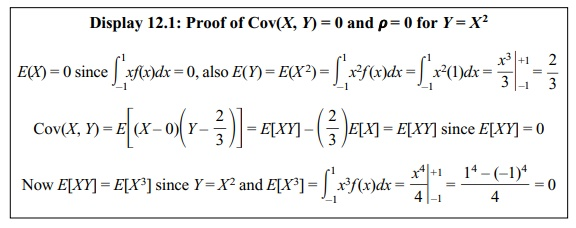# Pearson’s Product Moment Correlation Coefficient and Its Sample Estimate

| Home | | Advanced Mathematics |

## Chapter: Biostatistics for the Health Sciences: Correlation, Linear Regression, and Logistic Regression

The formulae for a Pearson sample product moment correlation coefficient (also called a Pearson correlation coefficient).

PEARSON’S PRODUCT MOMENT CORRELATION COEFFICIENT AND ITS SAMPLE ESTIMATE

The formulae for a Pearson sample product moment correlation coefficient (also called a Pearson correlation coefficient) are shown in Equations 12.1 and 12.2. The deviation score formula for r isWe will apply these formulae to the small sample of weight and height measure-ments shown in Table 12.2. The first calculation uses the deviation score formula (i.e., the difference between each observation for a variable and the mean of the variable).

The data needed for the formulae are shown in Table 12.3. When using the cal-culation formula, we do not need to create difference scores, making the calcula-tions a bit easier to perform with a hand-held calculator.

We would like to emphasize that the Pearson product moment correlation mea-sures the strength of the linear relationship between the variables X and Y. Two variables X and Y can have an exact non-linear functional relationship, implying a form of dependence, and yet have zero correlation. An example would be the func-tion y = x2 for x between –1 and +1. Suppose that X is uniformly distributed on [0, 1] and Y = X2 without any error term. For a bivariate distribution, r is an estimate of the correlation (ρ) between X and Y, where

ρ = Cov(X, Y) / [Var(X)Var(Y)]

The covariance between X and Y defined by Cov(X, Y) is E[(Xμx)(Yμy)], where μx and μy are, respectively, the population means for X and Y. We will show that Cov(X, Y) = 0 and, consequently, ρ = 0. For those who know calculus, this proof is shown in Display 12.1. However, understanding this proof is not essential to under-standing the material in this section.

TABLE 12.2. Deviation Score Method for Calculating r (Pearson Correlation Coefficient)TABLE 12.3. Calculation Formula Method for Calculating r (Pearson Correlation Coefficient)Display 12.1: Proof of Cov(X, Y) = 0 and ρ = 0 for Y = X 2